# Shannon sampling theorem

Every signal functionthat is band-limited to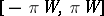for some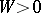can be completely reconstructed from its sample values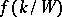, taken at nodes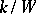,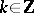, equally spaced apart on the real axis, in the form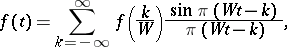the series being absolutely and uniformly convergent on. The latter series will be denoted by. Here, band-limited means thatcontains no frequencies higher thanor, in mathematical terms, thatis continuous, square-integrable on(i.e., of finite energy) and that its-Fourier transform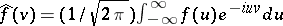vanishes outside(see [a13], [a10], p. 51, [a8], [a5], [a14]).

The theorem is also associated with the names of E.T. Whittaker, K. Ogura, V.A. Kotel'nikov, H.P. Raabe, and I. Someya.

Band-limited signals suffer under severe restrictions, since, by the Paley–Wiener theorem, they can be extended to an entire function on the whole complex plane. Thus, they cannot be simultaneously time-limited. However, both situations are covered by the so-called "approximate sampling" theorem, which is valid for not necessarily band-limited signals. It is due to J.R. Brown [a2] and states that if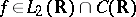and, then for the aliasing error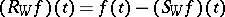one has the estimateso that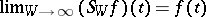uniformly in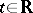. In particular, ifis band-limited to, then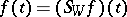for.

In essence, the sampling theorem is equivalent (in the sense that each can be deduced from the others) to five fundamental theorems in four different fields of mathematics. In fact, for band-limited functions the sampling theorem (including sampling of derivatives) is equivalent to the famous Poisson summation formula (Fourier analysis) and the Cauchy integral formula (complex analysis, cf. Cauchy integral theorem). Further, the approximate sampling theorem is equivalent to the general Poisson summation formula, the Euler–MacLaurin formula, the Abel–Plana summation formula (numerical mathematics), and to the basic functional equation for the Riemann zeta-function (number theory). The Poisson summation formula can also be interpreted as a trace formula [a15], p. 48. Two final connections are that the seriescan also be regarded as a limiting case of the Lagrange interpolation formula (as the number of nodes tends to infinity), while the Gauss summation formula of special function theory is a particular case of Shannon's theorem. See, e.g., [a7], [a4] and the references therein.

There are several types of errors that may influence the accuracy of the reconstruction of a signal function from its sample values; namely, the truncation error, which arises if only a finite number of samples is taken into account, the amplitude error, also called quantization error, which arises when instead of the exact valuesonly approximate values are available, and the time jitter error, which arises when the sample points are not met correctly but might differ by some. All such errors (including the aliasing error) can occur in combination (see, e.g., [a8]).

Sampling theory can be put in an abstract setting, in which the band-limited functionis represented by a sampling series of the form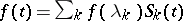, where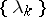is a discrete subset of the domain ofand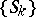is a set of appropriate "reconstruction functions" forming a basis or frame for a suitable function space (most often, a Hilbert space with reproducing kernel; cf. also Hilbert space). The methods give some unification of the approaches, and facilitate connections with important principles of sampling theory, including the Nyquist–Landau minimal rate for stable sampling, and sets of stable sampling, interpolation or uniqueness. The approach is flexible, and applies to multi-band (i.e., the support of the Fourier transform is a union of several disjoint intervals), multi-channel (i.e., the samples are not all taken fromitself, but also from a transformed version of), and multi-dimensional sampling. See [a10].

The first study of sampling and reconstruction in an abstract harmonic analysis setting is due to I. Kluvánek (1965). He replaced the time domainby a general locally compact Abelian group, and the frequence domain,again, by the dual group. Instead of sampling at regularly distributed points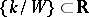, a function defined onis now sampled at the points of a discrete subgroup of. See [a1].

When the Fourier transform is replaced by the Mellin transform, with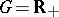, one is led to the exponential sampling theorem of N. Ostrowski and others (1981); see [a6].

How to Cite This Entry:
Shannon sampling theorem. P.L. Butzer (originator), Encyclopedia of Mathematics. URL: http://encyclopediaofmath.org/index.php?title=Shannon_sampling_theorem&oldid=11459
This text originally appeared in Encyclopedia of Mathematics - ISBN 1402006098